# Making the Best of Things

A Very Useful Thing About Slope Formulas

As you have worked through the previous posts and exercises, I hope you have noticed something special about places on a graph where the derivative is zero.  These places have horizontal tangent lines.  And in their own neighborhoods, they are often a local maximum or local minimum.  This piece of information is very helpful when you are trying to solve an “optimization” problem.

For example…

Suppose you are firing a projectile on a horizontal surface at a fixed launch speed, v.  And let’s also suppose that air friction is negligible.  What angle will maximize the range (horizontal distance) of the projectile?

You may remember the answer from your first year of physics.  It is intuitive and there was a way to get the answer without calculus.  But we can still use this example to illustrate the calculus method.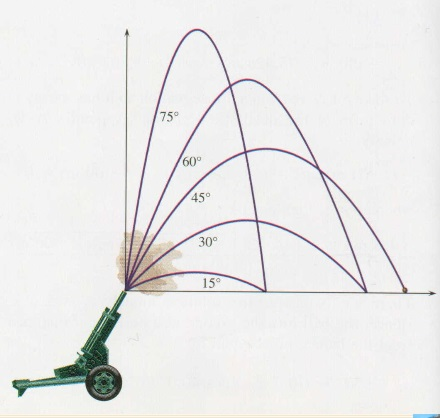(Graphic from http://outreach.phas.ubc.ca/phys420/p420_00/darren/web/range/range.html where you can also see a review of the projectile physics)

STEP 1: Express the quantity to be optimized as a function of a single variable.

In this case, a review of that website or last year’s notes will remind you that the distance varies with the launch angle by the rule: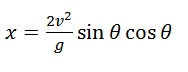In brief, we resolved the launch velocity into components, used the vertical component to determine time in air and then multiplied that time by the horizontal component of the velocity.  But now that we have this expression, for our current purposes it would be helpful to make use of the double angle identity for the sine function.  We can write: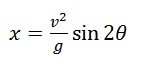Don’t worry if you would not have remembered how to do all that without notes.  The point is to learn what we can do with this expression now that we have it.

STEP 2: Find the derivative

This is one we know how to do:  we know the derivative of sine functions and we know the special case of the chain rule.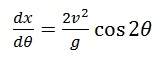STEP 3: What is the domain of interest to us?

When a math book asks for the domain of a function, they usually mean the largest, most inclusive domain possible.  For a sine function, that would be all real numbers.  But that is not what we are after here.  We need to think about the portion of the domain that is relevant.  To fire this cannonball as far as we can, clearly we will choose an angle between 0 and π/2 radians.

STEP 4: Where in that domain is the derivative equal to zero?

In this case…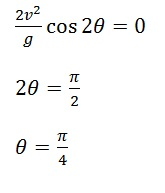(OK, call it 45 degrees if you prefer.)

STEP 5: (Your math teacher may not like this) Convince ourselves that we are done.

In math class, once you have found where the derivative is zero (or undefined), you are not nearly done.  You have to investigate each of these “critical points” to see what kind of extreme it is and to justify your conclusion with such things as sign charts and 2nd derivative tests.  But in physics class, you are going to get away a little easier because of the following claim:

If a “smooth” function has only one critical point on some interval and the value of the function is higher at that critical point than at either of the endpoints, then that value is the “absolute maximum” value.

So in the example at hand, we know the cannonball’s range is zero when the angle is zero, and its zero when the angle is 90 degrees (as the ball goes straight up and down).  But it is NOT zero when the launch angle is 45 degrees.  But the tangent line is horizontal at 45 degrees.  So that’s the “best” angle.

You still have to learn to do things the math-class way.  There are lots of functions with multiple critical points.  And there are functions that have horizontal tangent lines at places that turn out not to maxima or minima.  So you do have to know how to investigate further.

But the problems you will see in AP Physics don’t turn out that way.  Most often, there will be only one critical point and it will be the one we were looking for.  Here is another example I have always liked.Suppose you have a large cylindrical tank full of water, open at the top.  You are going to punch a hole in the tank somewhere along its side and you want the resulting jet of water to land as far from the tank as possible.  Where should you make the hole?  And where will that initial jet of water land?

Let’s say you make the hole y units from the ground and the initial jet lands x units from the tank.

STEP 1: Determine x as a function of y

First we need the “speed of efflux” which you can think of as the horizontal launch velocity of the water coming out of the hole.  There is a neat result from fluid dynamics that shows that the water leaves the tank at the same speed as if it had free-fallen from the top of the tank.  It’s called Torricielli’s Law.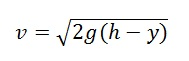http://en.wikipedia.org/wiki/Torricelli’s_law  (where I also got the graphic above)

Now, we just find the time in air and multiply by that launch speed.

For time in air, we can use the fact that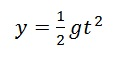and solve for time to get: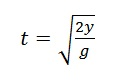So for the distance as a function of the height of the hole we get: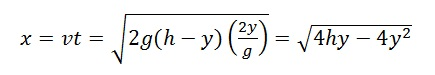STEP 2: Find the derivative

Well, it seems we need the more advanced version of the chain rule. But there is a trick to make things easier.  The distance x will be at its maximum when the term inside the square root is at its maximum.  So let’s ignore the square root and just maximize the innards.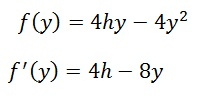STEP 3: Domain of interest?  We can’t make a hole below the ground or above the height of the cylinder so we only have to consider y values between 0 and h.

STEP 4: Set the derivative equal to zeroWe need a hole in the middle!

STEP 5:  Convince ourselves that we are done

There was only one critical point.  And the endpoints both lead to a distance of zero.  So the hole at y=h/2 is the one that gives us the farthest landing point.

And what is that landing point? Substitute y=h/2 into our original function, which was:I will leave the substitution and clean-up to you.  I will say that it cleans up quite nicely and has a memorable result.  The first of my students to post the correct result in a comment wins some silly thing or other.

Quick Recap and Closing Remarks

In AP Physics, when you want to find the maximum or minimum possible value of some quantity, most of the time it will just be a matter of taking a derivative, then setting it equal to zero and then waving your hands around to convince us that you are done.

The hardest part of the process is not going to be the calculus.  It’s going to be setting up the variables and finding the expression for the quantity you hope to optimize.  That’s why you had all those homework problems back in pre-calc that said “Express this thing in terms of that thing”.

I guess I should mention that if you are having trouble either finding the derivative or solving after you set it equal to zero, you may find your TI82 to be of some use.  But honestly, sometimes it feels like the calculator only gives intelligible answers to people who already know what the solution looks like.

## One thought on “Making the Best of Things”

1.Dan Gulko on said:

I am leaving a comment for the answer to the simplification of the landing point. The answer is absolute value of h. Do I win some silly thing or other?